Function Repository Resource:

# SudokuGraph

Generate an n-Sudoku graph

Contributed by: Alejandra Ortiz
 ResourceFunction["SudokuGraph"][n] generates an undirected graph with n4 vertices corresponding to the cells of an n2x n2 sudoku board and with edges corresponding to all pairs of cells that belong to the same row, column, or block of the puzzle.

## Details

ResourceFunction["SudokuGraph"] takes the same options as Graph.

## Examples

### Basic Examples (1)

The first few sudoku graphs on differently sized sudoku boards:

 In:=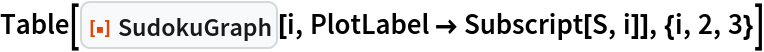Out=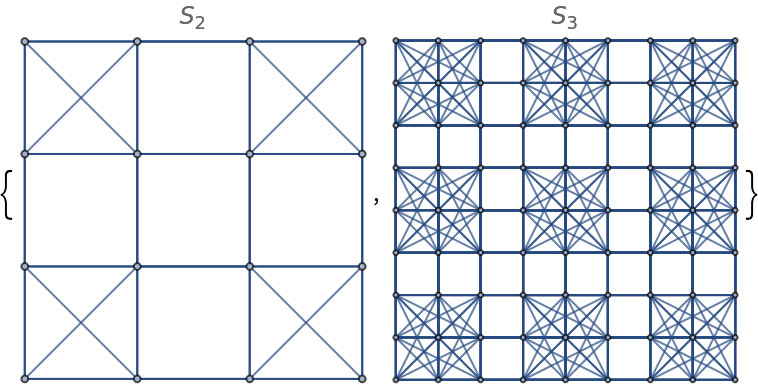### Scope (1)

The Sudoku graph is automatically laid out in the order of the cell numeration on the Sudoku board:

 In:=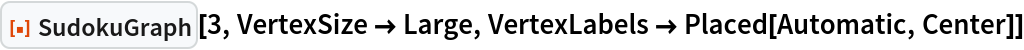Out=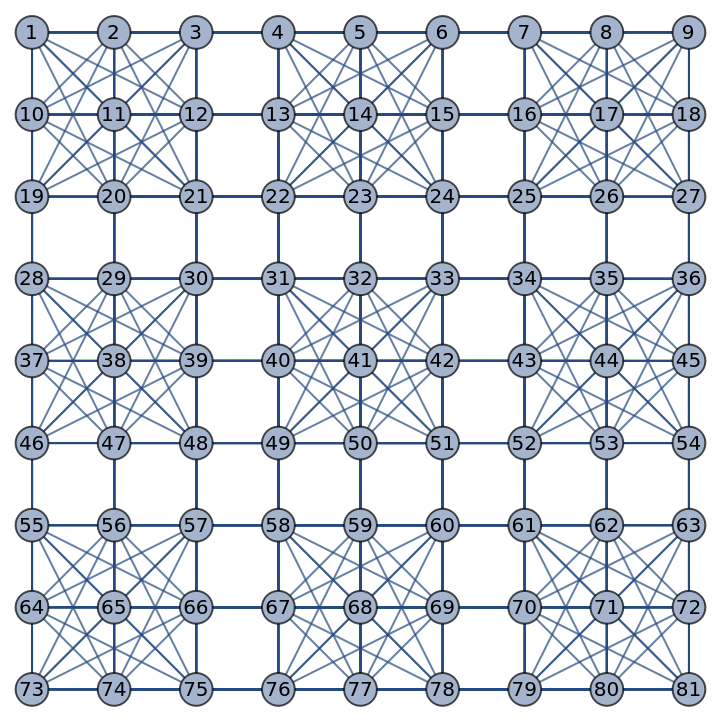### Options (6)

#### GraphHighlight (2)

Highlight the vertex 1:

 In:=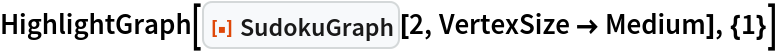Out=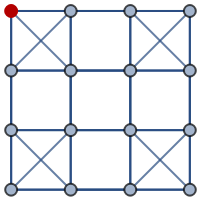Highlight the edge 16:

 In:=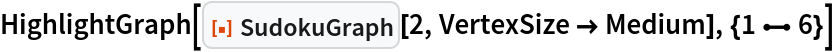Out=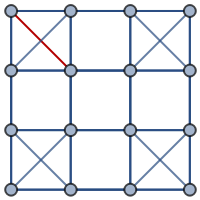#### VertexLabels (2)

Label individual vertices:

 In:=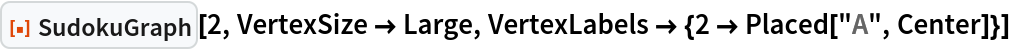Out=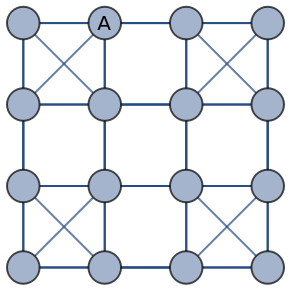Label all vertices:

 In:=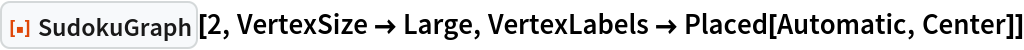Out=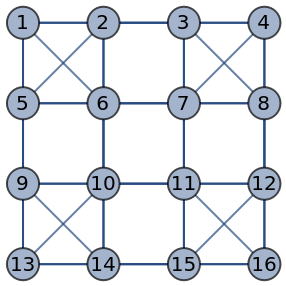#### VertexSize (2)

By default, the size of vertices is computed automatically:

 In:=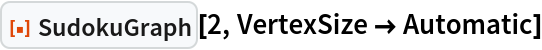Out=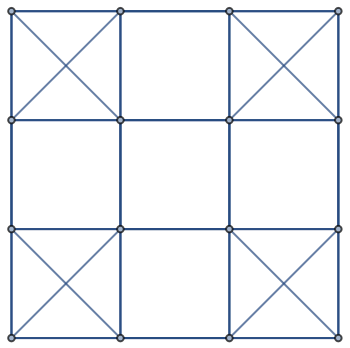Specify the size of all vertices using symbolic vertex size:

 In:=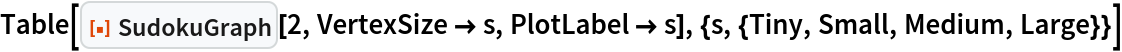Out=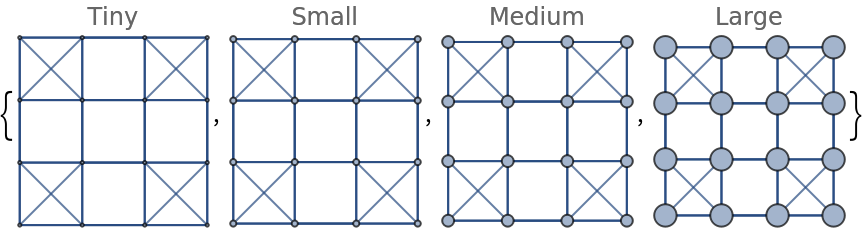### Applications (1)

Find a vertex coloring of a sudoku graph:

 In:=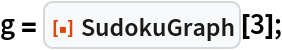In:=Out=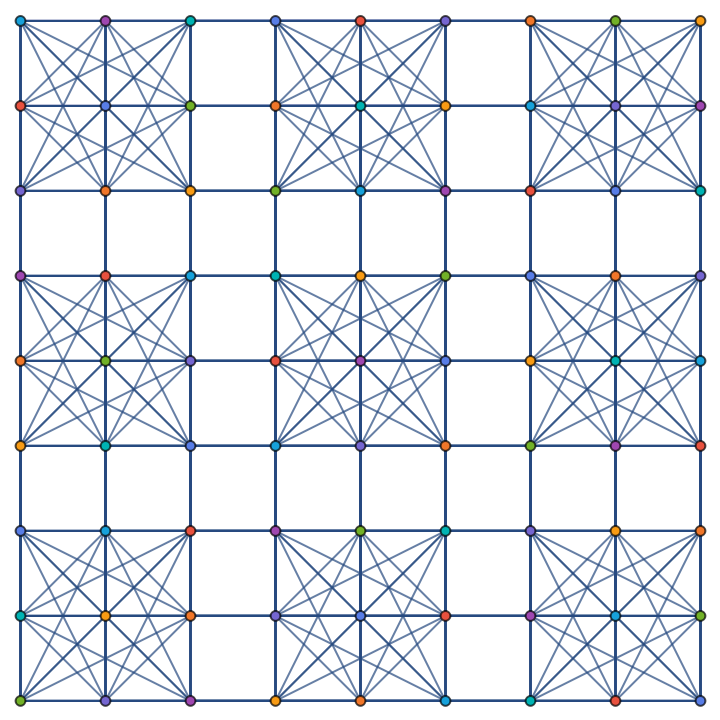## Publisher

Wolfram Summer School

## Version History

• 1.0.0 – 14 June 2023